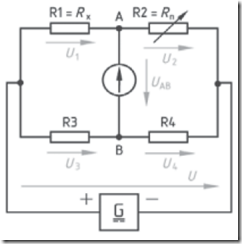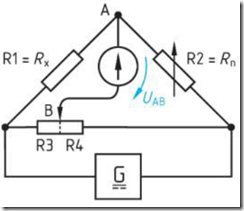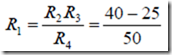Home > English > Wheatstone Bridge

# Wheatstone Bridge

Development of series resistor series and paralelmenghasilkan principle of the Wheatstone Bridge. DC voltage source circuit dole empatbuah resistor. R1 series with R2, and R3 in series with a voltage Kirchoff R4.Hukum stating the amount of voltage drop equal to the voltage source.Figure 8.10 Wheatstone Bridge

AB point mounted voltmeter to measure the voltage difference, if the meter shows zero, meaning that the voltage U1 = U3 is called a balanced condition. If U1 ≠ U3 called unbalanced condition and the meter showed specific figures. The practical application used models Figure 5.63, where R1 = Rx is a prisoner who sought magnitude. R2 = Rn is the resistance that can be arranged magnitude. R3 and R4 of shearing resistance. Shearing resistance by adjusting the position B, until the voltmeter zero position. This condition is called equilibrium, then apply the formula of equilibrium Wheatstone bridge.Figure 8.11 Bridge Wheatsone

Example:
Wheatstone bridge, note the value of R2 = 40 Ω, 25 Ω = R3, R4 = 50 Ω. Calculate the amount of R1 in a state of equilibrium!
If UAB = 0 V, then"Tasikmalaya, Indonesia"